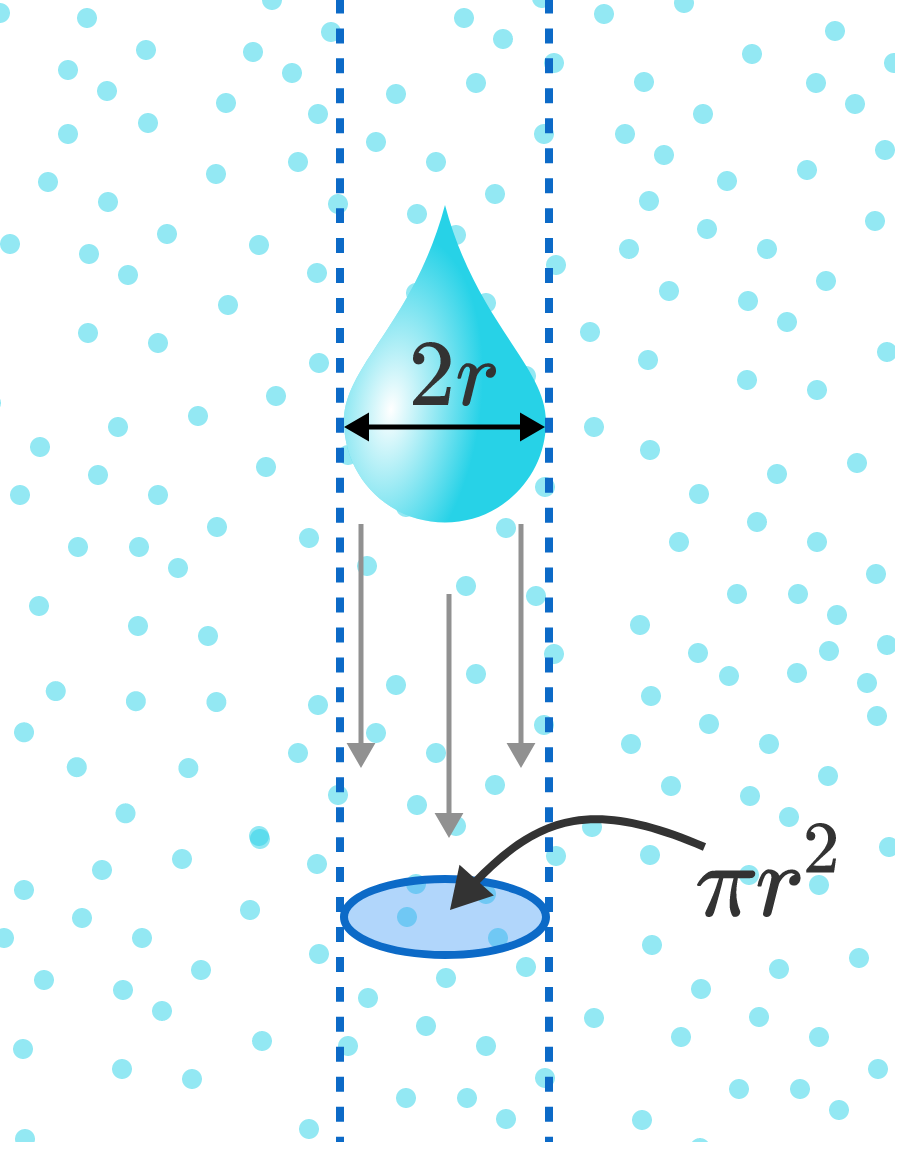# Small RaindropConsider the following model:

A raindrop falls from rest with a small initial mass, $m_0.$ As it falls, it accumulates mass by absorbing the smaller water drops in its path, which we approximate by the uniform density $\rho_\textrm{atm}.$

Throughout the fall, it maintains the same characteristic shape defined by its width, $2r,$ which increases as it picks up mass at the rate $\dot{m} = \rho_\textrm{atm}\pi r^2 v$ (in time $dt$, it sweeps out a vertical cylinder of height $vdt$).

How will its velocity depend on time? Surprisingly, this complicated problem has a simple solution: after a short time, the raindrop will accelerate at the constant rate $a=\frac gK$ (convince yourself of this!).

What is the value of $K?$


Details and Assumptions:

• Neglect the effects of air resistance, wind speed, the density of air, or any other atmospheric factor except those stated in the problem.

Inspired by a similar problem by Tapas Mazumdar

×